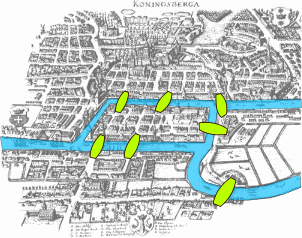# Seven Bridges of Königsberg facts for kids

Kids Encyclopedia FactsMap of Königsberg in Euler's time showing the actual layout of the seven bridges, highlighting the river Pregel and the bridges

The Seven Bridges of Königsberg is a historically famous problem in mathematics. Leonhard Euler solved the problem in 1735. This led to the beginning of graph theory. This then led to the development of topology.

The city of Königsberg in Prussia (now Kaliningrad, Russia) was set on both sides of the Pregel River. It included two large islands which were connected to each other and the mainland by seven bridges.

The problem was to find a way to walk through the city by crossing each bridge once and only once. The islands could not be reached by any route other than the bridges. Every bridge must have been crossed completely every time. The walk need not start and end at the same spot. Euler proved that the problem has no solution.

## Euler's analysis

First, Euler pointed out that the choice of route inside each land mass does not matter. The only important property of a route is the order in which the bridges are crossed. So, he changed the problem to abstract terms. This laid the foundations of graph theory. He removed all features except the list of land masses and the bridges connecting them. In the language of graph theory, he replaced each land mass with an abstract "vertex" or node. Then he replaced each bridge with an abstract connection, an "edge". An edge (road) recorded which two vertices (land masses) were connected. In this way, he formed a graph.

The graph drawn is an abstract picture of the problem. So, the edges can be joined in any way. Only whether two points are connected or not are important. Changing the picture of the graph does not change the graph itself.

Next, Euler observed that (except at the endpoints of the walk), whenever one enters a vertex by a bridge, one leaves the vertex by a bridge. In any walk of the graph, the number of times one enters a vertex equals the number of times one leaves it. If every bridge has been crossed exactly once, it follows that, for each land mass (except for the ones chosen for the start and finish), the number of bridges touching that land mass must be even. This is because if there are n bridges, it is crossed exactly 2n times. However, all four of the land masses in the original problem are touched by an odd number of bridges (one is touched by 5 bridges, and each of the other three are touched by 3). There are at most two masses which can be the endpoints of a walk. So the proposition of a walk crossing each bridge once leads to a contradiction.

In modern language, Euler shows that whether a walk through a graph crossing each edge once is possible or not depends on the degrees of the nodes. The degree of a node is the number of edges touching it. Euler shows that a necessary condition for the walk is that the graph be connected and have exactly zero or two nodes of odd degree. This result stated by Euler was later proved by Carl Hierholzer. Such a walk is now called an Eulerian path or Euler walk. If there are nodes of odd degree, then any Eulerian path will start at one of them and end at the other. Since the graph representing the historical Königsberg has four nodes of odd degree, it cannot have an Eulerian path.

Euler's work was presented to the St. Petersburg Academy on August 26, 1735. It was published as Solutio problematis ad geometriam situs pertinentis (The solution of a problem relating to the geometry of position) in the journal Commentarii academiae scientiarum Petropolitanae in 1741. It is available in English in The World of Mathematics.

## Importance in the history of mathematics

In the history of mathematics, Euler's solution of the Königsberg bridge problem is considered to be the first theorem of graph theory. Graph Theory is a subject now generally regarded as a branch of combinatorics.

## Present state of the bridges

Two of the seven original bridges were destroyed during the bombing of Königsberg in World War II. Two others were later demolished. They were replaced by a modern highway. The three other bridges remain, although only two of them are from Euler's time (one was rebuilt in 1935). Thus, as of 2000, there were five bridges in Kaliningrad.

In terms of graph theory, two of the nodes now have degree 2, and the other two have degree 3. Therefore, an Eulerian path is now possible, but since it must begin on one island and end on the other, it is impractical for tourists.

## Images for kidsSeven Bridges of Königsberg Facts for Kids. Kiddle Encyclopedia.